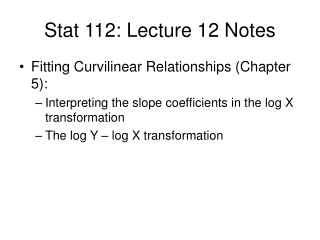DownloadDownload PresentationStat 112: Lecture 12 Notes

# Stat 112: Lecture 12 Notes

Télécharger la présentation## Stat 112: Lecture 12 Notes

- - - - - - - - - - - - - - - - - - - - - - - - - - - E N D - - - - - - - - - - - - - - - - - - - - - - - - - - -
##### Presentation Transcript

1. Stat 112: Lecture 12 Notes • Fitting Curvilinear Relationships (Chapter 5): • Interpreting the slope coefficients in the log X transformation • The log Y – log X transformation

2. Log Transformation

3. Interpreting the Coefficient on Log X

4. Log Transformation of Both X and Y variables • It is sometimes useful to transform both the X and Y variables. • A particularly common transformation is to transform X to log(X) and Y to log(Y)

5. Heart Disease-Wine Consumption Data (heartwine.JMP)

6. Evaluating Transformed Y Variable Models The log-log transformation provides slightly better predictions than the simple linear regression Model.

7. Interpreting Coefficients in Log-Log Models

8. Another interpretation of coefficients in log-log models

9. Another Example of Transformations: Y=Count of tree seeds, X= weight of tree

10. By looking at the root mean square error on the original y-scale, we see that Both of the transformations improve upon the untransformed model and that the transformation to log y and log x is by far the best.

11. Prediction using the log y/log x transformation • What is the predicted seed count of a tree that weights 50 mg? • Math trick: exp{log(y)}=y (Remember by log, we always mean the natural log, ln), i.e.,